Journal of Industrial Engineering and Management

On the exact calculation of the mean stock level in the base stock periodic review policy

Eugenia Babiloni, Manuel Cardós, Ester Guijarro

Universitat Politècnica de València (SPAIN)

Accepted May 2011

Babiloni, E., Cardós, M., & Guijarro, E. (2011). On the exact calculation of the mean level in the base stock periodic review policy. Journal of Industrial Engineering and Management, 4(2), 194-205. doi:10.3926/jiem.2011.v4n2.p194-205

---------------------

Abstract:

Purpose: One of the most usual indicators to measure the performance of any inventory policy is the mean stock level. In the generalized base stock, periodic review policy, the expected mean stock during the replenishment cycle is usually estimated by practitioners and researchers with the traditional Hadley-Whitin approximation. However it is not accurate enough and exact methods suggested on the related literature focus on specific demand distributions. This paper proposes a generalized method to compute the exact value of the expected mean stock to be used when demand is modelled by any uncorrelated, discrete and stationary demand pattern.

Design/methodology/approach: The suggested method is based on computing the probability of every stock level at every point of the replenishment cycle for which it is required to know the probability of any stock level at the beginning of the cycle and the probability transition matrix between two consecutive periods of time. Furthermore, the traditional Hadley-Whitin approximation is compared with the proposed exact method over different discrete demand distributions

Findings: This paper points out the lack of accuracy that the Hadley-Whitin approximation shows over a wide range of service levels and discrete demand distributions.

Research limitations/implications: The suggested method requires the availability of appropriate tools as well as a sound mathematical background. For this reason, approximations to it are the logical further research of this work.

Practical implications: The use of the Hadley-Whitin approximation instead of an exact method can lead to underestimate systematically the expected mean stock level. This fact may increase total costs of the inventory system.

Originality/value: The original derivation of an exact method to compute the expected mean stock level for the base stock, periodic review policy when demand is modelled by any discrete function and backlog is not allowed.

Keywords: mean stock level, periodic review, discrete demand distribution

---------------------

# 1      Introduction and related literature

The control procedure of the traditional periodic-review, base stock policy consists on examining the status of an item every R fixed time periods and launching a replenishment order which raise the inventory position (on-hand stock plus on-order stock minus backorders) to the order up to level S. This policy is commonly denoted by (R, S) policy. In this paper, we focus on the exact estimation of the expected mean on-hand stock level in the (R, S) policy when demand is modelled by any discrete distribution function. For a more easy reading of the paper the on-hand stock or physical stock will be refer just as stock further on.

In practice, an inventory policy is designed to satisfy a predefined target service level, such as the cycle service level or the fill rate, whereas the mean stock level is minimized. Another approach consists on the minimization of total costs of the systems, but even then, an accurate enough estimation of the mean stock level is necessary. Traditionally, the estimation of the mean stock level in the (R, S) policy has been fulfilled by using the easy-to-compute Hadley-Whitin approximation, which derivation can be found in Hadley and Whitin (1963). This approximation is computing by means of the following the expression (1).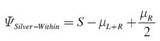(1)

where L is the replenishment lead time and µR+L and µR represents the mean demand during R+L and L consecutive periods respectively.

Apart from the Hadley-Whitin approximation, there is a handful of works that are dedicated to derive exact methods to estimate the mean stock level in the (R, S) policy. Nevertheless, they apply only under specific demand distributions, such as the Hadley and Whitin (1963) method that apply only when demand is Poisson distributed; the Nahmias and Smith (1994) method that calculates the mean stock level based on the two parameters of a negative binomial demand distribution in a multilevel inventory system; or the van der Heijden and de Kok (1992) method that applies for compound Poisson demand process with gamma distributed customers. This method also appears in van der Heijden and de Kok (1998) where the performance of the traditional Hadley-Whitin approximation is assessed for this particular demand context showing that it can be very inaccurate, especially for low customer service levels.

To the best of our knowledge, no authors have actually suggested a generalized and exact method to compute the mean stock level able to be applied when demand is modelled by using any discrete distribution function. Note that exact methods referenced above apply only under specific demand conditions. Furthermore we assume that unsatisfied demands are lost which is the most common situation in the retail sector as Johansen (2005) points out.

Therefore, this paper has a twofold objective: (1) to derive an exact method to compute the mean stock level when demand process is stationary, independent and identically distributed (i.i.d.) and modelled with any discrete distribution function; and (2) to point out the lack of accuracy the Hadley-Whitin approximation shows under several discrete demand distributions and over a wide range of service levels.

This paper is organized as follows. Section 2 dedicates to describing the basic assumptions and the derivation of the exact method to compute the expected mean stock level. Section 3 shows the comparison between the exact and the Hadley-Whitin approximation of the mean stock level followed by the discussion of the results. Finally conclusions and further research are briefly pointed out in Section 4.

# 2      Exact calculation of the mean stock level in the periodic review policy

As known, the (R, S) policy place replenishment orders every R fixed time periods which is received L time periods after being launched. Figure 1 shows an example of the evolution of the on-hand stock in a periodic review system.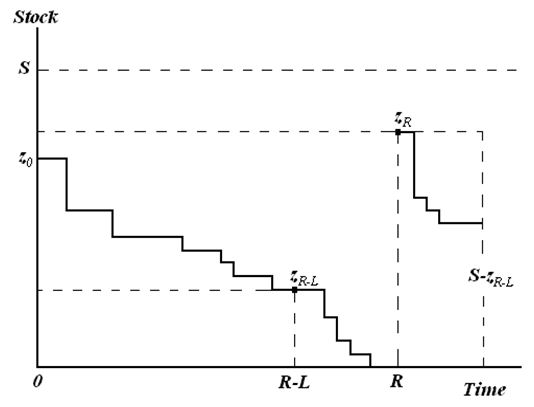Figure 1. “Example of the stock evolution in a periodic review system”.

The mathematical derivation of the proposed method is based on the following assumptions: (i) the time is discrete and is organized in a numerable and infinite succession of equi-spaced instants; (ii) L is constant; (iii) backordering is not allowed and therefore L<R; (iv) the replenishment order is added to the inventory at the end of the period in which the order is received; (v) demand during a period is fulfilled with the inventory at the beginning of that period; and (vi) the demand process is assumed to be stationary with a known, discrete and i.i.d. distribution function. Notations used in this paper are listed as follows:

 S = order up to level, R = review period and replenishment cycle corresponding to the time between two consecutive deliveries, L = lead time for the replenishment order, zt = on-hand stock at time t from the first reception, Dt = accumulated demand during t consecutive periods of time, D[t,  t-1] = demand that occurs between t and n consecutive periods of time, µt = mean demand during t periods of time, ft(·) = probability density function of demand at time t, Ft(·) = cumulative distribution function of demand during t periods of time, Yt = expected stock level at time t, Y = expected mean stock level during the replenishment cycle.

In general, the expected stock level at any time of the cycle depends on the probability of every feasible stock value. Then obviously: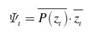(2)

where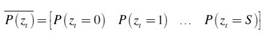and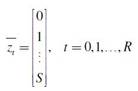Therefore, the expected mean stock level during the replenishment is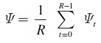(3)

However, the problem lies in computing the probability vector of every stock level in each period of the cycle,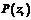. Note that the probability of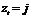depends on the stock level at the precious period,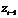and on the demand that appears between two consecutive periods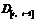. Then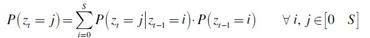(4)

where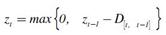(5)

Expressing (4) as matrixes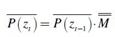(6) Where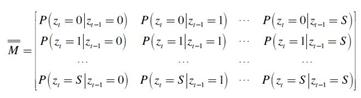(7)

is defined as the probability transition matrix between stock levels of two consecutive periods and according to (5):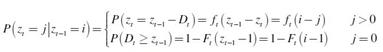(8)

Therefore it is possible to compute the probability of every stock level at any point of the cycle if we know the probability of every feasible stock level at the beginning of the cycle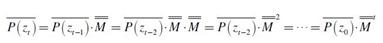(9)

And therefore, the expected mean stock level during the replenishment cycle is calculated by using the following expression: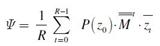(10)

that can be applied to any discrete, stationary and i.i.d. demand pattern and leads to the exact value of the mean stock level.

The estimation of the probability of every stock level at the beginning of the cycle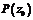can be obtained following the approach suggested by Cardós, Miralles, and Ros (2006).

# 3      Experimentation

3.1 Experiment desing

This section is dedicated to compare the exact method and the traditional Hadley-Whitin approximation in order to analyse deviations which arise from using the latest. For that purpose, we design an experiment that follows two steps: (1) Given a known discrete demand distribution and a target cycle service level, CSL, we compute the base stock that guarantees reaching the target; and (2) with the base stock from step 1, we compute the value of the mean stock level using the expression (10) and the Hadley-Whitin approximation (expression (1)).

In general, the selection of the most appropriate discrete distribution function of demand is a difficult task. Syntetos and Boylan (2006) recommend using the Negative Binomial distribution, Teunter, Syntetos, and Babai (2010) suggest using a compound Bernoulli distribution, Janssen, Heuts, and de Kok (1998) and Strijbosch, Heuts, and van der Schoot (2000) proposes using a Compound Bernoulli Distribution, whereas Silver, Pyke, and Peterson (1998) recommend the Poisson distribution whenever the item is considered strategic but slow moving. In keeping with this, we have selected the following discrete distributions: (1) Bernoulli(p); (2) Binomial(n, p); and (3) Poisson(l). Taking into account that Bernoulli distribution is equivalent to Binomial distribution with n=1, the experiment considers the Binomial and the Poisson distribution with the appropriate set of parameters. The parameters of each distribution are selected to meet the smooth and the intermittent demand pattern suggested by Syntetos, Boylan, and Croston (2005). Regarding the parameters of the inventory system, an extensive range of values are selected to consider as many different and realistic contexts as possible. Table 1 summarizes the parameters and values used in the experiment which was programmed in Java.

The feasible combination of values of Table 1 leads to 19.866 different cases. The estimating errors arising from estimating the mean stock level using the      Hadley-Whitin approximation instead or the exact method are analyzed by using the relative error expressed in terms of per 100, by means of the following expression: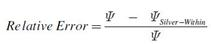(11)

Note that computing relative errors as in expression (11) enables not only to know the magnitude of the error but also the type of error by analyzing its sign. A negative relative error means that the approximation overestimates the expected mean stock. Otherwise, if the relative error is positive, the approximation is underestimating the real mean stock level.

 Factor Values Demand distribution Binomial (n, p) n= 1,2,3,4,5,6,7,8,9,10,15 p= 0.1,0.25,0.5,0.75,0.8,0.9,0.99 Poisson (l) l= 0.01,0.1,0.5,1,2,3,5,7,10 Cycle service level, CSL 0.50,0.55,0.60,0.65,0.70,0.75,0.80,0.85,0.90,0.95,0.99 Inventory policy Review Period, R 2,3,4,5,10,15,20,30 Lead time, L 1,3,5,10

Table 1. “Experiment factors and values”.

3.2 Results and discussion

Table 2 shows the maximum, minimum, average and standard deviation, sorted by the target CSL, of the relative errors the Hadley-Whitin approximation fall into. The relative errors for every of the 19,866 cases will be available upon request. A first look into it reveals that the relative errors are always positive and therefore the Hadley-Whitin approximation tends to underestimate the mean stock level. In practice the underestimation of the expected mean stock has important consequences for the system since the stock level is above the expected and therefore, based on the Hadley-Whitin estimation, managers would plan wrongly its material and logistic requirement.

As expected, the Hadley-Whitin approximation improves its performance when the CSL increases. This result was pointed out by van der Heijden and de Kok (1998) for gamma distributed demands. However, we realize that average relative errors are not near to zero when the CSL is near 1. This fact is easier observable through Figure 2, where the relative errors from the 19,866 cases are represented versus the target CSL. We observe how the size of the relative errors decreases when the CSL increases. However, when CSL>0.8, the relative error per target CSL shows an almost similar pattern. Therefore we can affirm that the Hadley-Whitin approximation is biased and not an enough accurate approximation of the mean stock level, even if the target CSL is near to 1.

 CSL Number of cases Maximum relative error Minimum relative error Average relative error Standard deviation 0.50 1,806 100.00% 0.51% 21.43% 16.08% 0.55 1,806 80.99% 0.51% 18.77% 13.42% 0.60 1,806 65.61% 0.51% 16.20% 11.03% 0.65 1,806 64.03% 0.51% 14.64% 9.90% 0.70 1,806 56.33% 0.51% 12.75% 8.33% 0.75 1,806 36.24% 0.51% 11.38% 7.37% 0.80 1,806 35.37% 0.51% 10.15% 6.75% 0.85 1,806 32.67% 0.38% 9.05% 6.30% 0.90 1,806 32.67% 0.26% 8.10% 5.96% 0.95 1,806 32.67% 0.26% 7.20% 5.73% 0.99 1,806 32.67% 0.18% 6.36% 5.57%

Table 2. “Maximum, minimum, average and standard deviation of relative errors”.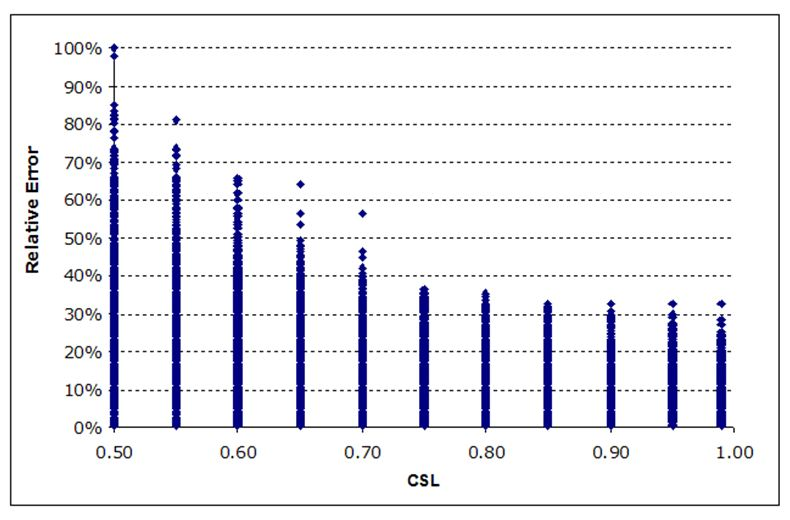Figure 2. “Relative errors versus the target CSL for the 19.866 resulted cases”.

# 4      Conclusion and further research

This paper derives an exact method to calculate the expected mean stock level suitable for any stationary, discrete, and i.i.d. demand pattern for the periodic review, base stock policy (R, S). The suggested exact method is based on computing the probability transition matrix of stock levels between two consecutive periods of time in the replenishment cycle. This method is appropriate for any discrete demand distribution and therefore it do not depend on parameters of the distribution.

Traditionally, the computation of the expected stock level has been done using the Hadley-Whitin approximation. However, this paper shows that: (1) it systematically underestimates the mean stock level and therefore it is biased, and (2) its accuracy decreases when the cycle service level decreases. Hence, in practice, using the Hadley-Whitin approximation could lead to fall into significant errors not only for the inventory system itself but also could influence decisions related with the purchase and production policy and as a result, to increasing the total costs of the system.

Further extensions of this work should be focused on applying the easy to compute estimation of the probability of every stock level at the beginning of the cycle suggested by Cardós and Babiloni (2011) in order to evaluate its performance when using it for the mean stock level estimation.

This paper is part of a wider research project devoted to identify the most simple and effective stock policy to properly manage any particular demand pattern based on the characteristics of demand itself.

Acknowledgements

The authors would like to thank the two anonymous referees their constructive comments on earlier versions of this paper.

References

Cardós, M., & Babiloni, E. (2011). Exact and approximate calculation of the cycle service level in periodic review inventory policies. International Journal of Production Economics, 161(1), 63-68.

Cardós, M., Miralles, C., & Ros, L. (2006). An exact calculation of the cycle service level in a generalized periodic review system. Journal of the Operational Research Society, 57(10), 1252-1255.
doi:10.1057/palgrave.jors.2602121

Hadley, G., & Whitin, T. (1963). Analysis of Inventory Systems. Englewood Cliffs, NJ: Prentice-Hall.

Janssen, F., Heuts, R., & de Kok, T. (1998). On the (R, s, Q) inventory model when demand is modelled as a compound Bernoulli process. European Journal of Operational Research, 104(3), 423-436.
doi:10.1016/S0377-2217(97)00009-X

Johansen, S. G. (2005). Base-stock policies for the lost sales inventory system with Poisson demand and Erlangian lead times. International Journal of Production Economics, 93-94, 429-437.

Nahmias, S., & Smith, S. A. (1994). Optimizing Inventory Levels in a Two-echelon Retailer System with Partial Lost Sales. Management Science, 40(5), 582-596.
doi:10.1287/mnsc.40.5.582

Silver, E. A., Pyke, D. F., & Peterson, R. (1998). Inventory Management and Production Planning and Scheduling. John Wiley & Sons.

Strijbosch, L. W. G., Heuts, R. M. J., & van der Schoot, E. H. M. (2000). A combined forecast - inventory control procedure for spare parts. Journal of the Operational Research Society, 51(10), 1184-1192.
doi:10.1057/palgrave.jors.2601013

Syntetos, A. A. & Boylan, J. E. (2006). On the stock control performance of intermittent demand estimators. International Journal of Production Economics, 103(1), 36-47.
doi:10.1016/j.ijpe.2005.04.004

Syntetos, A. A., Boylan, J. E., & Croston, J. D. (2005). On the categorization of demand patterns. Journal of the Operational Research Society, 56(5), 495-503.
doi:10.1057/palgrave.jors.2601841

Teunter, R. H., Syntetos, A. A., & Babai, M. Z. (2010). Determining order-up-to levels under periodic review for compound binomial (intermittent) demand. European Journal of Operational Research, 203(3), 619-624.
doi:10.1016/j.ejor.2009.09.013

van der Heijden, M. C., & de Kok, T. (1992). Customer waiting times in an (R, S) inventory system with compound Poisson demand. Mathematical Methods of Operations Research, 36(4), 315-332.
doi:10.1007/BF01416231

van der Heijden, M. C., & de Kok, T. (1998). Estimating stock levels in periodic review inventory systems. Operations Research Letters, 22(4-5), 179-182.
doi:10.1016/S0167-6377(98)00020-0

Journal of Industrial Engineering and Management, 2011

Article's contents are provided on a Attribution-Non Commercial 3.0 Creative commons license. Readers are allowed to copy, distribute and communicate article's contents, provided the author's and Journal of Industrial Engineering and Management's names are included. It must not be used for commercial purposes. To see the complete license contents, please